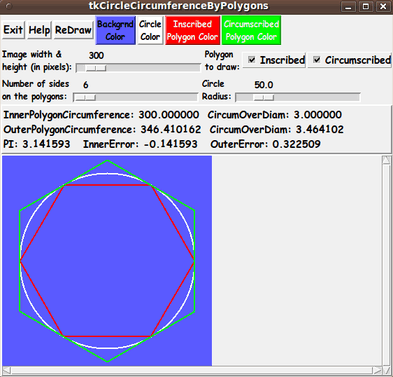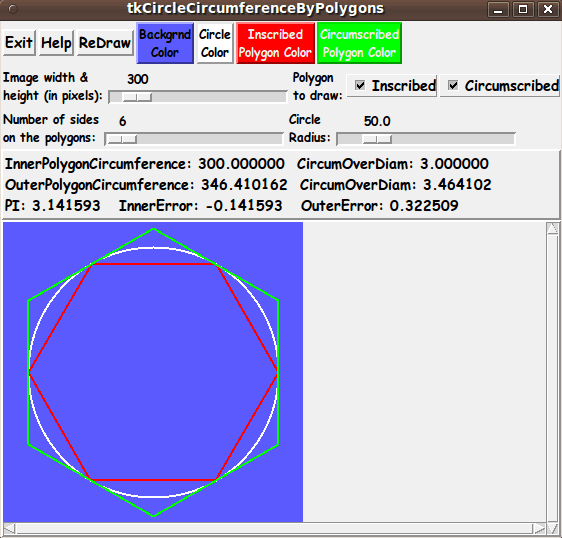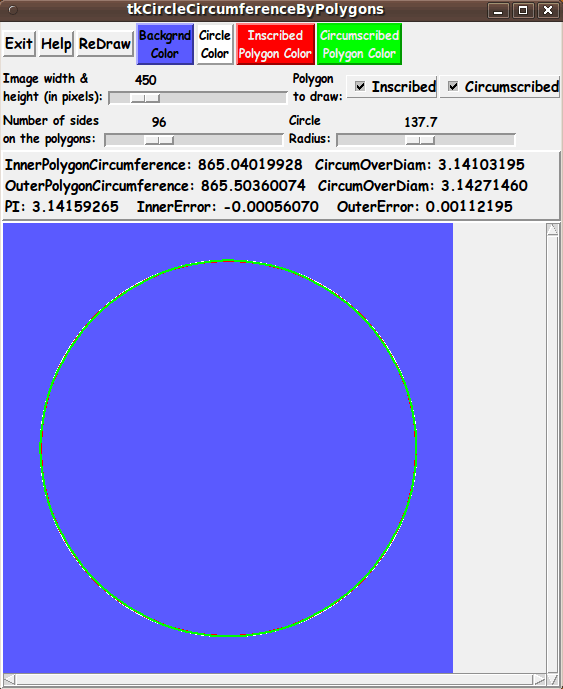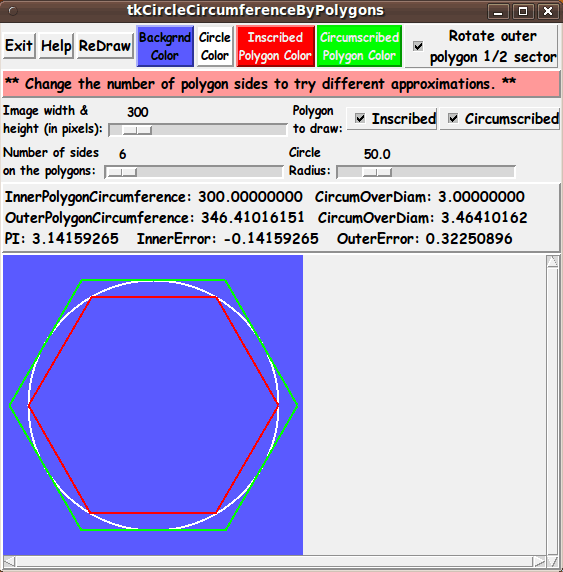## Tcl-Tk script 'tkCircleCircumference ByPolygons'

##### (FE = Freedom Environment)GUI interface to
approximate
the value of PI
with polygons
inscribing and
cirumscribing
a circle ---
like Archimedes did.

Large, hi-res images are below.

FE 'tkGooies' Description Page >

This
'tkCircleCircumferenceByPolygons'
tkGooie Code Page

``````

SQUARE-BRACKETS indicate a comment (not to be placed on the GUI).
BRACES          indicate a Tk 'button' widget.
A COLON         indicates that the text before the colon is on a 'label' widget.
<---O--->       indicates a Tk 'scale' widget.
CAPITAL-X       indicates a Tk 'checkbutton' widget.
CAPITAL-O       indicates a Tk 'radiobutton' widget (if any).
UNDERSCORES     indicate a Tk 'entry' widget (if any).

According to those conventions,
I created the following 'text sketch'.

FrameNames
VVVVVVVVVV
-----------------------------------------------------------------------------
tkCircleCircumferenceByPolygons
[window title]
-----------------------------------------------------------------------------

.fRbuttons   {Exit} {Help} {ReDraw} {Backgd  {Circle  {Inscribed     {Circumscribed
Color}   Color}   Polygon Color} Polygon Color}

50      3000
.fRcontrols1 Image width&height (pixels): <-----O----> Polygon to draw:  X Inner  X Outer

3          360            0.1            300.0
.fRcontrols2 Number of sides: <------O----->    Radius: <--------O--------->

.fRmsg       [ .......... Message line --- Calculated Circumferences are shown here .... ]

.fRcanvas    -----------------------------------------------------------------------------
|                                                                           A
|                                                                           |
|                                                                           |
|  'Canvas' for displaying the circle and polygons                          |
|   in a square image area.                                                 |
|                                                                           |
|                                                                           |
|                                                                           |
|                                                                           V
<-------------------------------------------------------------------------->

```
```

 Perhaps some aspects of the sketch could use a little more explanation:

``````

A LINE (HYPHENS or VERTICAL-BARS) WITH AN 'ARROW-HEAD' AT EACH END indicates
a Tk 'scale' widget --- or a 'scrollbar' widget.

A combination of VERTICAL-BAR CHARACTERS AND HYPHEN (or UNDERSCORE) CHARACTERS,
that outline a RECTANGULAR SHAPE, are used to indicate either a Tk 'canvas' or
a Tk 'listbox' widget or a Tk 'text' widget.

SCROLL-BAR 'ARROW-HEADS' (for a 'canvas', 'listbox', or 'text' Tk widget)
are drawn as follows:

UP    ARROW-HEAD   is drawn with a CAPITAL-A.
DOWN  ARROW-HEAD   is drawn with a CAPITAL-V.
LEFT  ARROW-HEAD   is drawn with a LESS-THAN sign.
RIGHT ARROW-HEAD   is drawn with a GREATER-THAN sign.

UP-and-DOWN    ARROW-HEADS  at the right/left of a box shape indicate
a VERTICAL SCROLL-BAR there.

LEFT-and-RIGHT ARROW-HEADS  at the bottom/top of a box shape indicate
a HORIZONTAL SCROLL-BAR there.

The arrow-heads on a horizontal scrollbar are joined by hyphens, rather than
underscores.

```
```

 From the diagram above, I could see that this GUI will contain about: 7 'button' widgets 4 'label' widgets 3 'scale' widgets 1 'canvas' widget (with x-y scrollbars) 2 'checkbutton' widgets 0 'radiobutton' widgets 0 'entry' widgets 0 'listbox' widgets 0 'text' widgets Assembling the pieces   (The GUI) Now it was a matter of putting the pieces together. I took 'code-pieces' from some of my other Tk scripts that 'draw' in an image area on a Tk canvas. I ended up with the following GUI as an initial display.Note that I have provided 4 color buttons on the GUI so that the user can control the background color of the image area the color of the circle outline the color of the 'inner' polygon outline the color of the 'outer' polygon outline I also provided several 'scale' widgets by which to control the size of the square image area (in pixels) the number of sides to use in drawing the 'inner' and 'outer' regular polygons a radius for the circle (in 'world coordinate units', not pixels) I also provided a couple of 'checkbutton' widgets on the GUI by which the user can choose to draw the 'inner polygon', the 'outer polygon', both, or neither. By default, the GUI starts with both polygons drawn over the circle --- and with hexagons for the two polygons. A message area in the middle of the GUI displays calculation results: circumference of the 'inner' polygon (in 'world units') circumference of the 'outer' polygon (in 'world units') ratio of these 2 circumferences to the diameter of the circle (which is twice the radius specified by the user) the difference of the two ratios from the 'true' value of pi --- Below is an image drawn after changing NumSides to 96 (like what Archimedes used) and increasing the size of the image area to 450 x 450 pixels --- and after changing the radius from 50.0 to 137.7.``````

0) Set general window & widget parms (win-name, win-position,
win-color-scheme, fonts, widget-geometry-parms, win-size-control).

1a) Define ALL frames (and sub-frames, if any).
1b) Pack   ALL frames and sub-frames.

2) Define & pack all widgets in the frames, frame by frame.
Within each frame, define ALL the widgets.
Then pack the widgets.

3) Define keyboard and mouse/touchpad/touch-sensitive-screen action
BINDINGS, if needed.

4) Define PROCS, if needed.

5) Additional GUI initialization (typically with one or more of
the procs), if needed.

```
```

 This Tk coding structure is discussed in more detail on the page A Canonical Structure for Tk Code --- and variations. This Tk coding structure makes it easy for me to find code sections --- while generating and testing this script, and when looking for code snippets to include in other scripts (code re-use). Experimenting with the GUI As in all my scripts that use the 'pack' geometry manager (which is all of my 100-plus Tk scripts, so far), I provide the four main 'pack' parameters: '-side' '-anchor' '-fill' '-expand' on all the 'pack' commands for the frames and widgets. I think I have found a good setting of the '-side', '-anchor', '-fill', and '-expand' parameters on the 'pack' commands for the various widgets of this GUI. In particular ... The 'canvas' widget will expand/contract appropriately when an image size 'scale' widget value is changed --- and button and label and scale widgets stay fixed in size and relative-location as the window size is changed. If anyone wants to change the way the GUI configures itself as the main window size is changed, they can experiment with the '-side', '-anchor', '-fill', and '-expand' parameters on the 'pack' commands for the various widgets --- to get the widget behavior that they want. --- Additional experimentation with the appearance of the GUI: You could change the fonts used for the various GUI widgets. For example, you could change '-weight' from 'bold' to 'normal' --- or '-slant' from 'roman' to 'italic'. Or change font families. In fact, you may NEED to change the font families, because the families I used may not be available on your computer --- and the default font that the 'wish' interpreter chooses may not be very pleasing. Furthermore, there are variables used to set geometry parameters of widgets --- parameters such as border-widths and padding. And you could change the '-relief' values for frames and widgets. Feel free to experiment with those 'appearance' parameters as well. Some features of the code There are plenty of comments in the code to describe what most of the code-sections are doing. The code 'comes together' in the 'Redraw' proc. See the comments in that proc to see how it is implemented. See the top of the 'PROCS' section for a list of the procs used in this Tk script. See comments in the procs for details on the purpose of each proc and for details on the methods by which each proc was implemented. Below is a quick overview of the procs --- to give an idea of the 'guts' of this utility:

``````

- setMappingVars_for_px2wc        - called by the 'set_MappingVars_forImageSquare'
proc below.

- set_MappingVars_forImageSquare  - called by the 'draw_*' procs below.

- Xwc2px                          - called by the 'draw_*' procs below.
- Ywc2px                          - called by the 'draw_*' procs below.

- draw_circle               - called by the 'Redraw' proc below.

- draw_inner_polygon        - called by the 'Redraw' proc below.

- draw_outer_polygon        - called by the 'Redraw' proc below.

- Redraw                    - called by the 'ReDraw' button.

- set_bkgd_color            - called by the background-color button '-command'

- set_circle_color          - called by the circle-color button '-command'

- set_inscribed_color       - called by the inner-polygon-color button '-command'

- set_circumscribed_color   - called by the outer-polygon-color button '-command'

- update_button_colors      - called by the 'set_*_color' procs and once
in the 'Additional GUI Initialization section.

- advise_user               - called by the 'Redraw' proc

- popup_msgVarWithScroll    - called by 'Help' button

```
```

 Some possible FUTURE ENHANCEMENTS There are quite a few enhancements that could be considered for this script, such as: Provide an XY plot (and an animation?) of the Pi estimates Instead of requiring the user to specify a single N (number of sides) at a time and click on the 'ReDraw' button for each calculation, we could add an 'Animate' or 'Automate' button on the GUI to run through the calculations for N = 3 to 1000 sides, say. The current image area could be used to display the circle and polygons --- or, since the polygons would rapidly blend into the circle --- the image area could be used to plot 'inner-polygon-circumference-over-diameter' and 'outer-polygon-circumference-over-diameter' against N. The XY plot would show the two ratios 'squeezing down' at the level of 3.1415... A 'WritePlot' button could be added to the GUI to allow for quickly writing out the plot to an image file --- such as a Postscript file. Alternatively, if it were desirable to show the XY plot being drawn, point (pair) by point (pair), the GUI could offer an option to write an animated-GIF file (or movie file) from a sequence of images generated from the animated XY plot. It would certainly be more convenient than having to do a bunch of image captures and manually contruct an animated-GIF (or movie) file from a sequence of still-image captures. I have implemented a Write-Ani-GIF option in several 'tkGooies' in the 'IMAGEtools' group, such as: wheeeMorph between two images I can use code from one or more of those Tk scripts to make it relatively easy to implement a Write-Ani-GIF option --- although I might have to do some sort of Postscript to GIF conversion. Different (Polygon) Methods of Calculating Pi There are many different methods of calculating pi --- many are series expansions or integrals --- but some also depend on using polygons --- such as the Liu Hui algorithm (described on Wikipedia). The current script and GUI are complex enough that it probably would not be advisable to add any more algorithms to this script. However, this script could be used as a model for implementing some of the other geometry-oriented methods of computing pi. 3D geometry algorithms There might be some nice algorithms for computing pi based on 3D geometry constructs. If one were ambitious, one could probably show the 3D geometry in an image area on a Tk canvas --- similar to the way that 3D model files can be read and viewed with a 3D viewer utility, like the FE 'tkGooies' Tk script at The bottom line here is that there are almost always non-trivial enhancements (or 'forks') that could be made to (or from) a Tk GUI 'app' like this. One advantage of this Tk script is that it is 'open' code --- available to anyone for enhancement. So if you would like to take a different approach to approximating pi, you are welcome to take this code and build on it. IN CONCLUSION As I have said on other code-donation pages on this site ... There's a lot to like about a utility that is 'free freedom' --- that is, no-cost and open-source so that you can modify/enhance/fix it without having to wait for someone else to do it for you (which may be never). A BIG THANK YOU to Ousterhout for starting Tcl-Tk, and a BIG THANK YOU to the Tcl-Tk developers and maintainers who have kept the simply MAH-velous 'wish' interpreter going.

 2016mar23 UPDATE --- RE-POSITIONING THE OUTER POLYGON The image of the inner and outer polygons (in many math books and web pages on Archimedes) is typically shown with the outer-polygon 'sectors' rotated from the inner-polygon sectors (by half of the polygon-sectors' angle). That is the configuration of the 2 polygons that was used in the initial Tk script implementation, as seen in the drawings in the images above. However, this 'rotated' configuration does not show that the inner-polygon sectors fit exactly into the outer-polygon sectors. Such a 'fitted' configuration would emphasize that, clearly, the lengths of the line-segments of the outer-polygon are longer than the line-segments of the inner polygon --- and thus that the circumference of the outer-polygon is always bigger than the circumference of the inner-polygon, for a given number of sides N. So I decided to add a 'checkbutton' widget to the top of the GUI, so that the user can draw the circle and 2 polygons with the 2 polygons aligned so that their corresponding sides are parallel. See the checkbutton --- and the new drawing configuration --- in the following image.This drawing configuration shows very convincingly that the circumference of the outer-polygon is always bigger than the circumference of the inner polygon, for any given N. Furthermore, it makes it easier to show, mathematically, that the two circumferences converge to each other. Let us compare the length of the segments of the inner and outer polygons when the sectors 'coincide'. Let 'r' be the radius of the circle, and 'R' be the distance to the end-points of the sides of the outer-polygon, from the center of the circle. Let N be the number of sides in the two polygons. Let ANG = 2*pi/N be the interior angle of the sectors. Let 'd' be the side-lengths of the inner-polygon. Let 'D' be the side-lengths of the outer-polygon. Then ...

``````

1) d = 2 * r * sin(ANG/2)

2) D = 2 * R * sin(ANG/2)

3) A diagram of an outer-polygon sector
and the definition of the cosine gives us
the relation:  r/R = cos(ANG/2)
So R = r/cos(ANG/2)

4) Equation-2 becomes D = 2 * r * sin(ANG/2)/cos(ANG/2)
= 2 * r * tan(ANG/2)

5) Hence
D - d = 2 * r * sin(ANG/2) * (1/cos(ANG/2) - 1)

So (D - d) approaches zero as ANG goes to zero.

Note that because BOTH of the 2 factors on the
far right go to zero as ANG goes to zero, the
difference (D - d) goes to zero 'quadratically'
(really fast) as ANG goes to zero.

```
```

 However, the question to be asked is: Does the difference in the CIRCUMFERENCES of the 2 polygons go to zero as ANG goes to zero?? The difference in circumferences, 'C' and 'c', say, is

``````

C - c = N*D - N*d

= N * (D - d)

= N * 2 * r * sin(ANG/2) * (1/cos(ANG/2) - 1)

where ANG = 2*pi/N.

```
```

 Note that as N is increasing, the last two factors are decreasing to zero. Some arguments using power series approximations to sin(ANG/2) show that sin(ANG/2) approaches ANG/2 as ANG goes to zero. So the N factor in front cancels with the N in the denominator of ANG = 2*pi/N --- and we are left with some constants times (1/cos(ANG/2) - 1), which goes to zero as ANG goes to zero. (This argument can be expressed even more rigorously --- using the definitions and proof techniques that are used in math classes on limits of sequences of numbers. "For any given positive number 'epsilon', no matter how small, there exists an integer 'N', such that for all 'n' greater than 'N', etc. etc.") So ... YES ... (C - c) does go to zero as ANG goes to zero --- and the two circumferences DO converge to the same number. That is, the upper-limit of the little-c's and the lower-limit of the big-C's is the same number. We are using here 'boundedness' theorems from math of numeric sequences: An increasing sequence that is bounded above converges to a finite limit. A decreasing sequence that is bounded below converges to a finite limit. We just showed above, fairly rigorously, by algebraic and trigonometric means, that the 2 limits must be the same number. In contrast, if we kept calculating the circumferences for larger and larger N, we could become more and more convinced that the two circumferences are approaching each other very closely. But the possiblity exists that the differences could 'level out' toward some very small number greater than zero. The argument above indicates, quite convincingly, that that will not happen. --- Note that whichever of the two 'rotated' positions you use for the outer polygon, all the results of computations for the circumferences and ratios and errors --- in the 'results' area of the GUI --- remain the same, for a given N. And the results are the same, for a given N, no matter the radius we choose for the circle --- via the radius 'scale' widget on the GUI. I have replaced the code at the CODE-link above with the code that provides the rotate-checkbutton.

Bottom of this web page for
presenting Tcl-Tk code for
'tkCircleCircumferenceByPolygons'
--- a 'tkGooie' script 'app'
in the FE 'tkGooies' system,
in the 'MATHed' subgroup of
the 'MATHtools' group.

OR ...

##### < Go to Top of Page, above. >

Page history:

This FE web page was created 2016 Mar 16.

Page was updated 2016 Mar 23.
(Added a 'rotate' checkbutton to the GUI.)

Page was updated 2016 Mar 28.